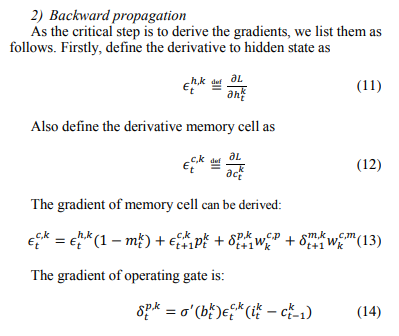# Custom RNN GMU implementation

Hi,
So there is a journal about other RNN besides LSTM and GRU and i want to implement it in Pytorch,

this is forward propagation formulaalso backward formulaand this is my implementation in Pytorch.

``````class JitGMUCell(jit.ScriptModule):

def __init__(self, input_size, hidden_size):
super(JitGMUCell, self).__init__()

self.hidden_size = hidden_size

self.weight_ih = nn.Parameter(torch.Tensor(3 * hidden_size, input_size))
self.weight_hh = nn.Parameter(torch.Tensor(3 * hidden_size, hidden_size))

self.weight_ch_m = nn.Parameter(torch.Tensor(hidden_size))
self.weight_ch_o = nn.Parameter(torch.Tensor(hidden_size))

self.bias_ih = nn.Parameter(torch.Tensor(3 * hidden_size))
self.bias_hh = nn.Parameter(torch.Tensor(3 * hidden_size))

self.reset_parameter()

@jit.ignore(drop=True)
def reset_parameter(self):
stdv = 1.0 / math.sqrt(self.hidden_size)
for weight in self.parameters():
nn.init.uniform_(weight, -stdv, stdv)

@jit.script_method
def forward(self, x, state):
# type: (Tensor, Tuple[Tensor, Tensor]) -> Tuple[Tensor, Tuple[Tensor, Tensor]]
hx, cx = state

xh = (
torch.mm(x, self.weight_ih.t()) + self.bias_ih +
torch.mm(hx, self.weight_hh.t()) + self.bias_hh
)

i, m, o = xh.chunk(3, 1)

m = m + (self.weight_ch_m * cx)
o = o + (self.weight_ch_o * cx)

i = torch.tanh(i)
m = torch.sigmoid(m)
o = torch.sigmoid(o)

# Base on Formula
h = (1 - m) * cx + (m * i)
c = (1 - o) * i + (o * cx)

return h, (h, c)
``````

my question is:

1. is my implementation are correct for those case (forward and backward)?
2. since the cell state not connected to hidden state, how can i do backprop on cell state?
3. even when using jit it has an error because cell state not connected to hidden state. one of the trick is to connect calculation with hidden state like `h = h + (c * 0)` but i don’t know it is the right way todo or not. is it okay to do that?

Can anybody point me how to achieve desired backprop like in formula in Pytorch (｡•́︿•̀｡).

Thank you, any response will be appreciate.